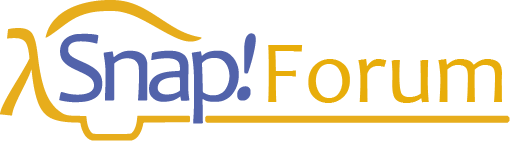# View on Snap!Con

## Abstract:

Snap_!_ 6.0 includes hyperblocks, reporters that normally expect single numbers or words as input but can now also accept vectors (simple lists) or matrices (lists of simple lists), or even higher-dimensional arrays, and report similarly shaped results.

The idea behind this new feature is 58 years old. It comes from the book A Programming Language by Kenneth Iverson, describing a language that came to be known by the initials of the book title: APL. Conceived as a computer language that would emulate the notation mathematicians use on chalkboards. Arrays are first class data in APL, and operations such as adding vectors and multiplying matrices are built in. Although arrays of numbers are the main emphasis, arrays of characters are also first class and can be used in the same ways.

In this workshop you will program in APL, getting to know its notoriously terse syntax and appreciating how that notation supports typical APL programming idioms. For example, make the array of coefficients in the polynomial f(x) = 4x^3 - 7x^2 + 5x + 3:

EXPR←4 ‾7 5 3

The overbar is for a negative number; no punctuation is needed for a vector. Then we can compute f(22):

((⍴EXPR)⍴22)⊥EXPR

The expression ρEXPR finds the length of EXPR, which is one more than the degree of the polynomial; in this example, it's 4. 4ρ22 makes four copies of 22. And the ⊥ operator finds the weighted sum of the numbers in EXPR with a weight of 1 for the rightmost number, and each weighting factor to the left is the previous one multiplied by the corresponding value from the left operand, all of which are 22 in our case.

24 60 60 ⊥ 5 4 3 computes the number of seconds in five hours, four minutes, and three seconds.

To try out APL online, visit
http://juergen-sauermann.de/try-GNU-APL